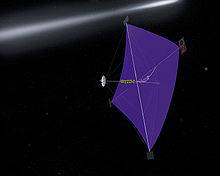# Can we use the momentum of light to propagate spacecrafts in space?

as we know light has momentum so theoretically we can use it but is it practical?
(also this is it that light only exerts force if incident on something?)

berkeman
Mentor
https://en.wikipedia.org/wiki/Solar_sail#### Attachments

anorlunda
Staff Emeritus
as we know light has momentum so theoretically we can use it but is it practical?
(also this is it that light only exerts force if incident on something?)

I would say "actual" rather than "practical", but the hydrogen bomb is triggered by use of light's momentum.
•